Select Page

# Number theory seminar

St. Petersburg State University and Euler International Mathematical Institute are happy to announce a new Number Theory seminar, headed by Eric Mortenson. To participate in the seminar, join zoom channel 310-172-1994, password: the number of quadratic non-residues modulo 23.

### Robert Osburn

Generalized Fishburn numbers, torus knots and quantum modularity

The Fishburn numbers are a sequence of positive integers with numerous combinatorial interpretations and interesting asymptotic properties. In 2016, Andrews and Sellers initiated the study of arithmetic properties of these numbers. In this talk, we discuss a generalization of this sequence using knot theory and the quantum modularity of the associated Kontsevich-Zagier series.

The first part is joint work with Colin Bijaoui (McMaster), Hans Boden (McMaster), Beckham Myers (Harvard), Will Rushworth (McMaster), Aaron Tronsgard (Toronto) and Shaoyang Zhou (Vanderbilt) while the second part is joint work with Ankush Goswami (RISC).

Presentation slides

### Amanda Folsom

Almost harmonic Maass forms and Kac-Wakimoto characters

We explain the modular properties of certain characters due to Kac and Wakimoto pertaining to sl(m|n)^, where n is a positive integer. We prove that these characters are essentially holomorphic parts of new automorphic objects we call “almost harmonic Maass forms,” which generalize both harmonic Maass forms and almost holomorphic modular forms. By using new methods involving meromorphic Jacobi forms, this generalizes prior works of Bringmann-Ono and Bringmann-Folsom, which treat the case n=1.  This is joint work with Kathrin Bringmann (University of Cologne).

Presentation slides

### George Andrews

How Ramanujan May Have Discovered the Mock Theta Functions

The mock theta functions made their first appearance in Ramanujan’s last letter to Hardy. Ramanujan explains that he is trying to find functions apart from theta functions that behave like theta functions near the unit circle. Where did he ever get the idea that such functions might exist? Why in the world would he consider the special q-series that he lists in his last letter? The object of this talk is to provide a plausible explanation for the discovery of mock theta functions.

Notes for the talk

### Lola Thompson

Counting quaternion algebras, with applications to spectral geometry

We will introduce some classical techniques from analytic number theory and show how they can be used to count quaternion algebras over number fields subject to various constraints. Because of the correspondence between maximal subfields of quaternion algebras and geodesics on arithmetic hyperbolic manifolds, these counts can be used to produce quantitative results in spectral geometry. This talk is based on joint work with B. Linowitz, D. B. McReynolds, and P. Pollack.

### Jeremy Lovejoy

Quantum q-series identities

As analytic identities, classical q-series identities like the Rogers-Ramanujan identities are equalities between functions for |q|<1. In this talk we discuss another type of q-series identity, called a quantum q-series identity, which is valid only at roots of unity. We note some examples from work of Cohen, Bryson-Ono-Pitman-Rhoades, and  Folsom-Ki-Vu-Yang, and then show how these and many more quantum identities follow from classical q-hypergeometric transformations.  In the second part of the talk we discuss examples of quantum q-series identities arising from knot theory.

### Martin Raum

Relations among Ramanujan-type Congruences

We present a new framework to access relations among Ramanujan-type congruences of a weakly holomorphic modular form. The framework is strong enough to apply to all Shimura varieties, and covers half-integral weights if unary theta series are available. We demonstrate effectiveness in the case of elliptic modular forms of integral weight, where we obtain a characterization of Ramanujan-type congruences in terms of Hecke congruences. Finally, we showcase concrete computer calculations, exploring the information encoded by our framework in the case of elliptic modular forms of half-integral weight. This leads to an unexpected dichotomy between Ramanujan-type congruences found by Atkin and by Ono, Ahlgren-Ono.

### Fernando Rodriguez-Villegas

Mixed Hodge numbers and factorial ratios

The factorial ratios of the title are numbers such as (30n)!n!/(6n)!(10n)!(15n)! considered by Chebyshev in his work on the distribution of prime numbers, which are integral for all n in a non-obvious way. I will discuss how integrality is related to the lack of interior points of the first few dilations of an associated polytope and the vanishing of certain Hodge numbers of associated varieties. This work is an offshoot of an ongoing project on hypergeometric motives joint with D.~Roberts and M.~Watkins.

Presentation slides

### Damaris Schindler

On the distribution of Campana points on toric varieties

In this talk we discuss joint work with Marta Pieropan on the distribution of Campana points on toric varieties. We discuss how this problem leads us to studying a generalised version of the hyperbola method, which had first been developed by Blomer and Bruedern. We show how duality in linear programming is used to interpret the counting result in the context of a general conjecture of Pieropan-Smeets-Tanimoto-Varilly-Alvarado.

Presentation slides

### Frank Garvan

A new approach to Dyson’s rank conjectures

In 1944 Dyson defined the rank of a partition as the largest part minus the number of parts, and conjectured that the residue of the rank mod 5 divides the partitions of 5n+4 into five equal classes. This gave a combinatorial explanation of Ramanujan’s famous partition congruence mod 5. He made an analogous conjecture for the rank mod 7 and the partitions of 7n+5. In 1954 Atkin and Swinnerton-Dyer proved Dyson’s rank conjectures by constructing several Lambert-series identities basically using the theory of elliptic functions. In 2016 the author gave another proof using the theory of weak harmonic Maass forms. In this talk we describe a new and more elementary approach using Hecke-Rogers series.

Presentation slides

### Matthias Beck

Partitions with fixed differences between largest and smallest parts

Enumeration results on integer partitions form a classic body of mathematics going back to at least Euler, including numerous applications throughout mathematics and some areas of physics. We study the number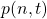of partitions of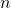with differencebetween largest and smallest parts. For example,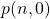equals the number of divisors of, the function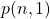counts the nondivisors of, and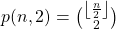. Beyond these three cases, the existing literature contains few results about, even though concrete evaluations of this partition function are featured in several entries of Sloane’s Online Encyclopedia of Integer Sequences.

Our main result is an explicit formula for the generating function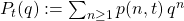. Somewhat surprisingly,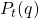is a rational function for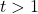; equivalently,is a quasipolynomial infor fixed(e.g., the above formula for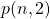is an example of a quasipolynomial with period 2). Our result generalizes to partitions with an arbitrary number of specified distances.

This is joint work with George Andrews (Penn State) and Neville Robbins (SF State).

Presentation slides

### Bruce Berndt

The Circle Problem of Gauss, the Divisor Problem of Dirichlet, and Ramanujan’s Interest in Them

Let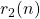denote the number of representations of the positive integeras a sum of two squares, and let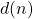denote the number of positive divisors of. Gauss and Dirichlet were evidently the first mathematicians to derive asymptotic formulas for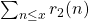and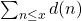, respectively, as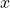tends to infinity. The magnitudes of the error terms for the two asymptotic expansions are unknown. Determining the exact orders of the error terms are the Gauss Circle Problem and Dirichlet’s Divisor Problem, respectively, and they represent two of the most famous and difficult unsolved problems in number theory.

Beginning with his first letter to Hardy, it is evident that Ramanujan had a keen interest in the Divisor Problem, and from a paper written by Hardy and published in 1915, shortly after Ramanujan arrived in England, we learn that Ramanujan was also greatly interested in the Circle Problem. In a fragment published with his Lost Notebook, Ramanujan stated two doubly infinite series identities involving Bessel functions that we think Ramanujan derived to attack these two famous unsolved problems. The identities are difficult to prove. Unfortunately, we cannot figure out how Ramanujan might have intended to use them. We survey what is known about these two unsolved problems, with a concentration on Ramanujan’s two marvelous and mysterious identities. Joint work with Sun Kim, Junxian Li, and Alexandru Zaharescu is discussed.

Presentation slides

### Ken Ono

Variations of Lehmer’s Conjecture on the Nonvanishing of the Ramanujan tau-function

In the spirit of Lehmer’s unresolved speculation on the nonvanishing of Ramanujan’s tau-function, it is natural to ask whether a fixed integer is a value of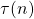, or is a Fourier coefficient of any given newform. In joint work with J. Balakrishnan, W. Craig, and W.-L. Tsai, the speaker has obtained some results that will be described here. For example, infinitely many spaces are presented for which the primes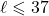are not absolute values of coefficients of any new forms with integer coefficients. For Ramanujan’s tau-function, such results imply, for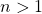, that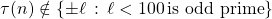.

Presentation slides